﻿ Spraying and burning of heptane - XSim

# Spraying and burning of heptane

OpenFOAM 4.x

## Case directory

\$FOAM_TUTORIALS/lagrangian/sprayFoam/aachenBomb

## Summary

We calculate the spontaneous ignition and combustion of liquid heptane (C7H16) when we sprayed it into air at a temperature of 800 K.Model geometry

Heptane is sprayed from position (0, 0.0995, 0) in the negative direction of the Y-axis for 0.00125 seconds. The gravity direction is in the negative direction of the Y-axis. The total mass, position, direction, duration, and velocity of the spray at each time are defined in the file "sprayCloudProperties" in the directory "constant" as "injectionModels" as follows.

```subModels
{
particleForces
{
sphereDrag;
}

injectionModels
{
model1
{
type            coneNozzleInjection;
SOI             0;
massTotal       6.0e-6;
parcelBasisType mass;
injectionMethod disc;
flowType        flowRateAndDischarge;
outerDiameter   1.9e-4;
innerDiameter   0;
duration        1.25e-3;
position        (0 0.0995 0);
direction       (0 -1 0);
parcelsPerSecond 20000000;
flowRateProfile table
(
(0              0.1272)
(4.16667e-05    6.1634)
(8.33333e-05    9.4778)
(0.000125       9.5806)
(0.000166667    9.4184)
(0.000208333    9.0926)
(0.00025        8.7011)
(0.000291667    8.2239)
(0.000333333    8.0401)
(0.000375       8.8450)
(0.000416667    8.9174)
(0.000458333    8.8688)
……
```

The reaction equation for combustion is "C7H16 + 11O2 → 7CO2 + 8H2O". The reaction equations are defined in the directory "chemkin" as the CHEMKIN format input files, and are used by importing them in the file "thermophysicalProperties" in the directory "constant".

The meshes are as follows, and the number of mesh is 168100.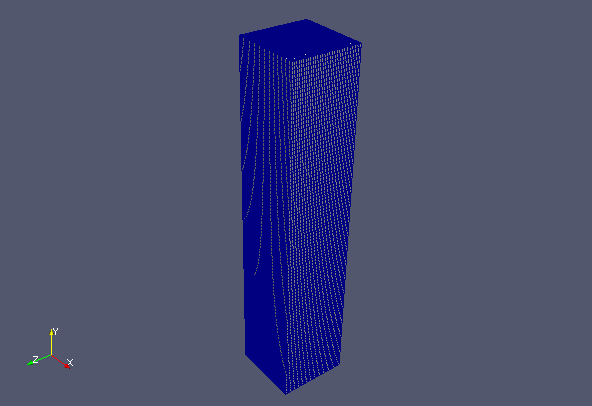Meshes

The calculation result is as follows.

First, here is the temperature in the center cross section of the analysis area. We can see how the heptane ignites near the spray area after blowing out and the fire spreads to the sprayed heptane.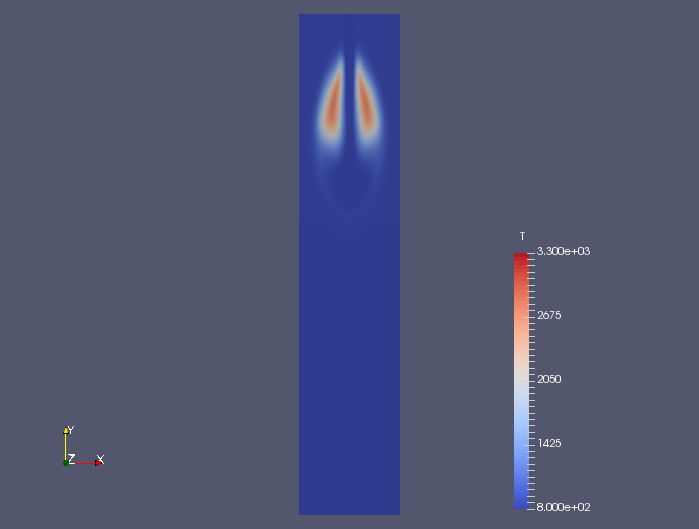Temperature at 0.00075 sec (T)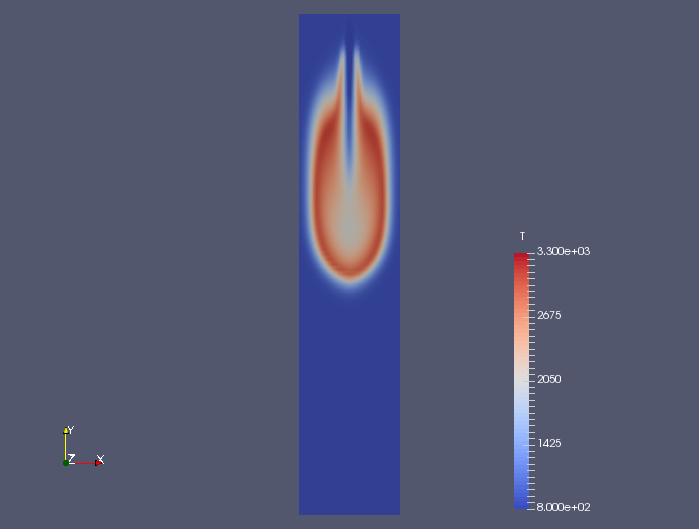Temperature at 0.00125 sec (T)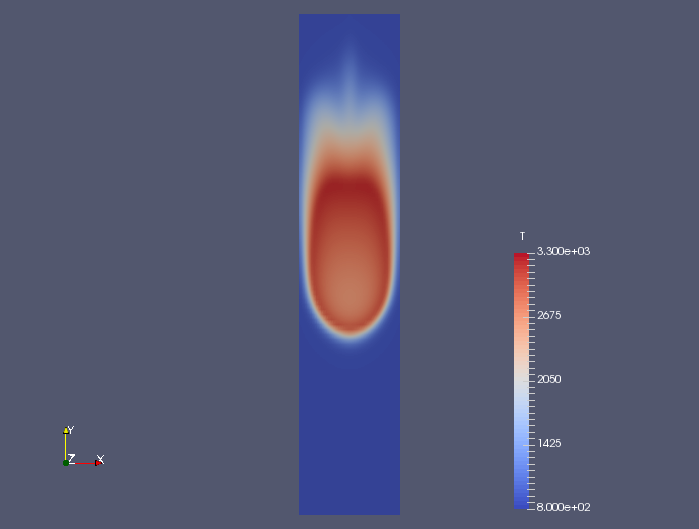Temperature at 0.0025 sec (T)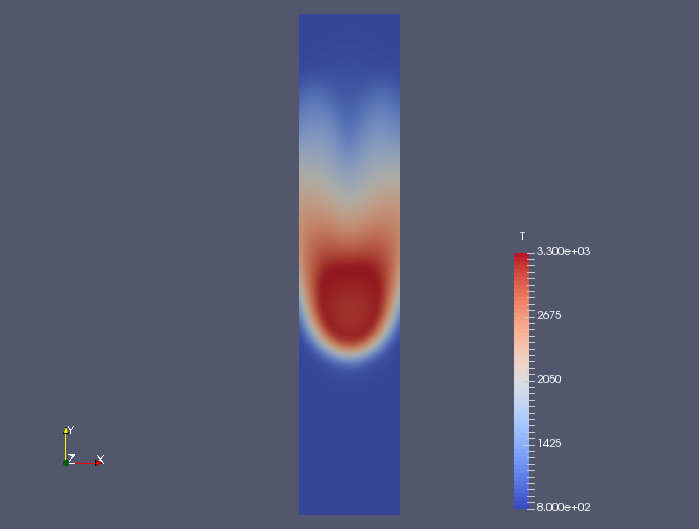Temperature at 0.005 sec (T)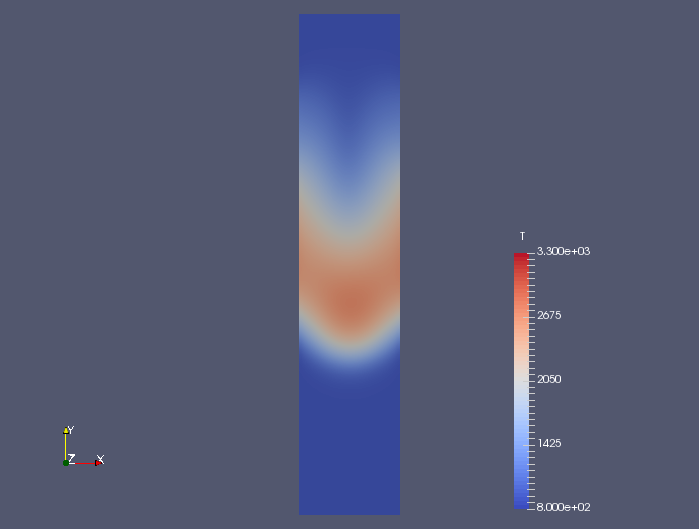Temperature at 0.01 sec (T)

Next is the flow velocity at the center cross section of the analysis area. The spray time is 0.00125 seconds, after which there is almost no flow.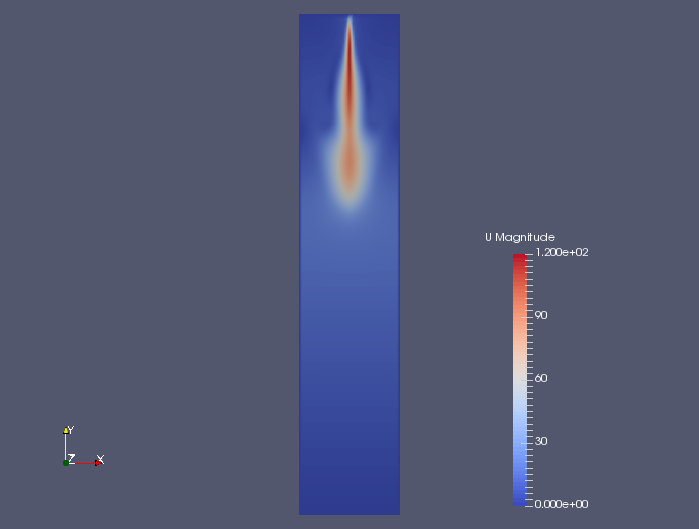Flow velocity at 0.00075 sec (U)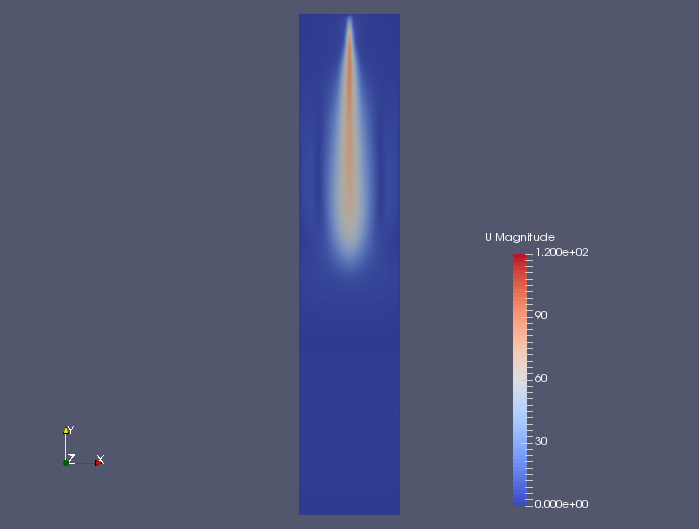Flow velocity at 0.00125 sec (U)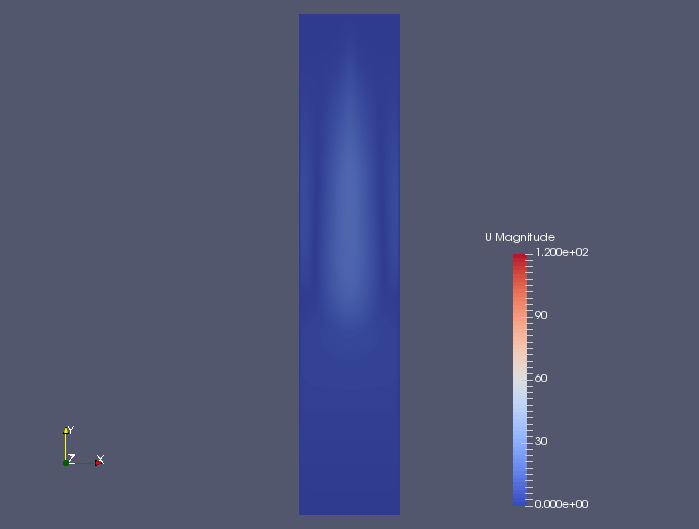Flow velocity at 0.0025 sec (U)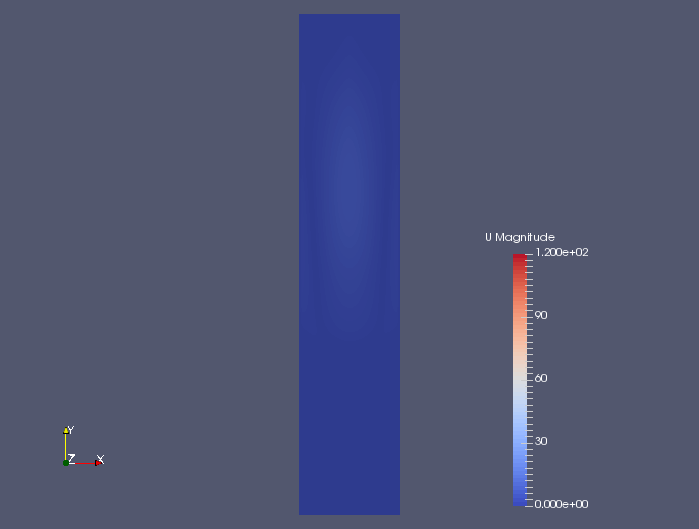Flow velocity at 0.005 sec (U)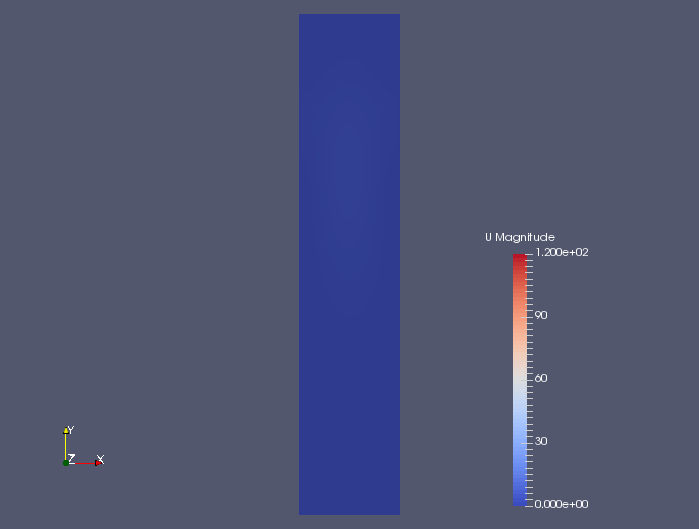Flow velocity at 0.01 sec (U)

## Commands

cp -r \$FOAM_TUTORIALS/lagrangian/sprayFoam/aachenBomb aachenBomb
cd aachenBomb

blockMesh
sprayFoam

paraFoam

## Calculation time

10 hours 34 minutes 44.4 seconds *Single, Inter(R) Core(TM) i7-2600 CPU @ 3.40GHz 3.40GHz